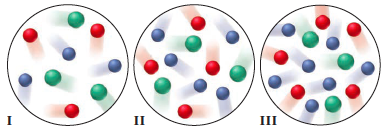# Problem: Three equal volumes of gas mixtures, all at the same  T, are depicted below (with gas A red, gas B green, and gas C blue):(c) In which sample, if any, do the gas particles have the highest average kinetic energy?

###### FREE Expert Solution

We can see from the samples that even though they have the same volume some samples have more particles than others.

We need to observe how the number of particles affects the average kinetic energy.

The average kinetic energy is proportional to the temperature. The equation relating the two is shown below:

86% (94 ratings)###### Problem Details

Three equal volumes of gas mixtures, all at the same  T, are depicted below (with gas A red, gas B green, and gas C blue):(c) In which sample, if any, do the gas particles have the highest average kinetic energy?